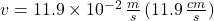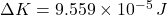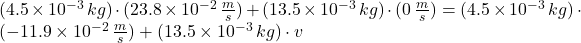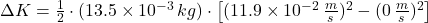## A 4.5 g coin sliding to the right at 23.8 cm/s makes an elastic head-on collision with a 13.5 g coin that is initially at rest. After the co

Question

A 4.5 g coin sliding to the right at 23.8 cm/s makes an elastic head-on collision with a 13.5 g coin that is initially at rest. After the collision, the 4.5 g coin moves to the left at 11.9 cm/s. (a) Find the final velocity of the other coin. 7.886 Incorrect: Your answer is incorrect. cm/s (b) Find the amount of kinetic energy transferred to the 13.5 g coin. 0.42 Incorrect: Your answer is incorrect. J

in progress 0
6 months 2021-08-09T07:36:44+00:00 2 Answers 4 views 0

(a) 11.9 cm/s or v’ = 0.119 m/s

(b) 9.56×10⁻⁵ J

Explanation:

(a)

From the law conservation of momentum,

total momentum before collision = Total momentum after collision

For elastic collision,

mu +m’u’ = mv+m’v’………………… Equation 1

Where m = mass of the first coin, u = initial velocity of the first coin, m’ = mass of the second coin, u’ = initial velocity of the second coin, v = final velocity of the first coin, v’ = final velocity of the second coin

Note: Since the second coin was initially at rest, u’ = 0 m/s, and m’u’ = 0

Therefore,

mu = mv+m’v’

make v’ the subject of the equation

v’ = (mu-mv)/m’……………………. Equation 2

Let: The right direction be positive and the left be negative.

given: m = 4.5 g, u = 23.8 cm/s, v = -11.9 cm/s (left) m’ = 13.5 g

Substitute into equation 2

v’ = [4.5×23.8-4.5×(-11.9)]/13.5

v’ = (107.1+53.55)/13.5

v’ = 160.65/13.5

v’ = 11.9 cm/s or v’ = 0.119 m/s

Hence the final velocity of the final velocity of the other coin = 0.119 m/s

(b)

The amount of kinetic energy transferred = 1/2m'(v’²-u’²)…………… Equation 3

Given: m’ = 13.5 g = 0.0135 kg, v’ = 0.119 m/s, u’ = 0 m/s (at rest)

Substitute into equation 3

The amount of kinetic energy transferred = 1/2(0.0135)(0.119²)

The amount of kinetic energy transferred = 0.00675(0.014161)

The amount of kinetic energy transferred = 9.56×10⁻⁵ J

a), b)Explanation:

a) The final velocity of the 13.5 g coin is found by the Principle of Momentum Conservation:The final velocity is:b) The change in the kinetic energy of the 13.5 g coin is: